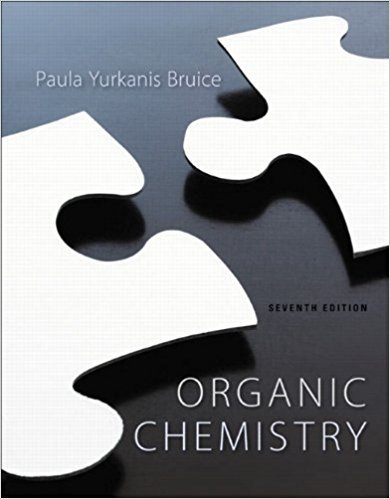×
×

# Solutions for Chapter 12: Organic Chemistry 7th Edition## Full solutions for Organic Chemistry | 7th Edition

ISBN: 9781269406772Solutions for Chapter 12

Solutions for Chapter 12
4 5 0 339 Reviews
15
2
##### ISBN: 9781269406772

Since 56 problems in chapter 12 have been answered, more than 160199 students have viewed full step-by-step solutions from this chapter. This textbook survival guide was created for the textbook: Organic Chemistry, edition: 7. This expansive textbook survival guide covers the following chapters and their solutions. Organic Chemistry was written by and is associated to the ISBN: 9781269406772. Chapter 12 includes 56 full step-by-step solutions.

Key Chemistry Terms and definitions covered in this textbook
• Acetal

A molecule containing two !OR or !OAr groups bonded to the same carbon

• acid

A substance that is able to donate a H+ ion (a proton) and, hence, increases the concentration of H+1aq2 when it dissolves in water. (Section 4.3)

Attraction between unlike molecules. (11.3)

• alkaloids

Naturally occurring amines isolated from plants.

• amphoteric oxide.

An oxide that exhibits both acidic and basic properties. (8.6)

• Beer’s law

In UV-Vis spectroscopy, an equation describing the relationship between molar absorptivity (e), absorbance (A), concentration (C), and path length (l): e = A (C Ž l)

• Bile acid

A cholesterol-derived detergent molecule, such as cholic acid, which is secreted by the gallbladder into the intestine to assist in the absorption of dietary lipids.

• Carboxylic acid

A compound containing a carboxyl, !COOH, group.

• Chlorofl uorocarbons (CFCs, Freons)

Compounds with one or two carbons, chlorine, and fl uorine, formerly used as refrigerants

• dispersion forces

Intermolecular forces resulting from attractions between induced dipoles. Also called London dispersion forces. (Section 11.2)

• electron density

A term associatedwith the probability of finding an electron in aparticular region of space.

• Fatty acid

A long, unbranched-chain carboxylic acid, most commonly of 12 to 20 carbons, derived from the hydrolysis of animal fats, vegetable oils, or the phospholipids of biological membranes.

• Fourier transform NMR (FT-NMR)

The modern NMR method that is based on a constant magnetic fi eld, a short pulse of electromagnetic radiation, and a mathematical Fourier transform to produce the spectrum

• lattice points

Points in a crystal all of which have identical environments. (Section 12.2)

• normal melting point

The melting point at 1 atm pressure. (Section 11.6)

• Oxidation

The loss of electrons. Alternatively, either the loss of hydrogens, the gain of oxygens, or both.

• phase diagram

A graphic representation of the equilibria among the solid, liquid, and gaseous phases of a substance as a function of temperature and pressure. (Section 11.6)

• proteins

Polypeptide chains comprised of more than 40 or 50 amino acids.

• solution

A mixture of substances that has a uniform composition; a homogeneous mixture. (Section 1.2)

• thioacetal

A compound that contains two SR groups, both of which are connected to the same carbon atom.

×